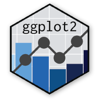Show Sidebar Hide Sidebar# geom_violin in ggplot2

How to make a density map using geom_violin. Includes explanations on flipping axes and facetting.

### New to Plotly?

Plotly's R library is free and open source!
You can set up Plotly to work in online or offline mode.
We also have a quick-reference cheatsheet (new!) to help you get started!

### Version Check

Version 4 of Plotly's R package is now available!
Check out this post for more information on breaking changes and new features available in this version.

``````library(plotly)
packageVersion('plotly')
``````
``````##  '4.9.1'
``````

### Basic violin plot

A basic violin plot showing how Democratic vote share in the 2018 elections to the US House of Representatives varied by level of density. A horizontal bar is added, to divide candidates who lost from those who won.

Source: Dave Wassermann and Ally Flinn for the election results and CityLab for its Congressional Density Index. Regional classifications are according to the Census Bureau.

``````library(plotly)
district_density <- read.csv("https://raw.githubusercontent.com/plotly/datasets/master/district_density.csv", stringsAsFactors = FALSE)
district_density\$cluster <- factor(district_density\$cluster, levels=c("Pure urban", "Urban-suburban mix", "Dense suburban", "Sparse suburban", "Rural-suburban mix", "Pure rural"))
district_density\$region <- factor(district_density\$region, levels=c("West", "South", "Midwest", "Northeast"))

p <- ggplot(district_density,aes(x=cluster, y=dem_margin, fill=cluster)) +
geom_violin(colour=NA) +
geom_hline(yintercept=0, alpha=0.5) +
labs(title = "Democratic performance in the 2018 House elections, by region and density",
x = "Density Index\nfrom CityLab",
y = "Margin of Victory/Defeat")

ggplotly(p)
``````

### Flipping the Axes

With geom_violin(), the y-axis must always be the continuous variable, and the x-axis the categorical variable. To create horizontal violin graphs, keep the x- and y-variables as is and add coord_flip().

``````library(plotly)
district_density <- read.csv("https://raw.githubusercontent.com/plotly/datasets/master/district_density.csv", stringsAsFactors = FALSE)
district_density\$cluster <- factor(district_density\$cluster, levels=c("Pure urban", "Urban-suburban mix", "Dense suburban", "Sparse suburban", "Rural-suburban mix", "Pure rural"))
district_density\$region <- factor(district_density\$region, levels=c("West", "South", "Midwest", "Northeast"))

p <- ggplot(district_density,aes(x=cluster, y=dem_margin, fill=cluster)) +
geom_violin(colour=NA) +
geom_hline(yintercept=0, alpha=0.5) +
labs(title = "Democratic performance in the 2018 House elections, by region and density",
x = "Density Index\nfrom CityLab",
y = "Margin of Victory/Defeat") +
coord_flip()

ggplotly(p)
``````